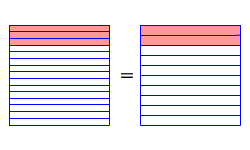# Converting Fractions to Decimals

To convert a fraction to a decimal, just divide the numerator by the denominator .

Example 1:

Write $\frac{3}{15}$ as a decimal.

Since $15$ is larger than $3$ , in order to divide, we must add a decimal point and some zeroes after the $3$ .  We may not know how many zeroes to add but it doesn’t matter.  If we add too many we can erase the extras; if we don’t add enough, we can add more.

$\begin{array}{l}\begin{array}{c}\\ 15\end{array}\begin{array}{c}\hfill 0.2\\ \hfill \overline{)3.0}\end{array}\\ \text{\hspace{0.17em}}\text{\hspace{0.17em}}\text{\hspace{0.17em}}\text{\hspace{0.17em}}\text{\hspace{0.17em}}\text{\hspace{0.17em}}\text{\hspace{0.17em}}\underset{_}{-3\text{\hspace{0.17em}}0\text{\hspace{0.17em}}\text{\hspace{0.17em}}}\\ \text{\hspace{0.17em}}\text{\hspace{0.17em}}\text{\hspace{0.17em}}\text{\hspace{0.17em}}\text{\hspace{0.17em}}\text{\hspace{0.17em}}\text{\hspace{0.17em}}\text{\hspace{0.17em}}\text{\hspace{0.17em}}\text{\hspace{0.17em}}\text{\hspace{0.17em}}\text{\hspace{0.17em}}\text{\hspace{0.17em}}\text{\hspace{0.17em}}0\end{array}$

So $\frac{3}{15}=0.2$ .

Remember that a decimal is really just a special way of writing a fraction that has a power of ten as a denominator. What we're doing here is rewriting $\frac{3}{15}$ as $\frac{2}{10}$ .Sometimes, you may get a repeating decimal .

Example 2:

Write $\frac{5}{6}$ as a decimal.

$\begin{array}{l}\begin{array}{c}\\ 6\end{array}\begin{array}{c}\hfill 0.8333\\ \hfill \overline{)5.0000}\end{array}\\ \text{\hspace{0.17em}}\text{\hspace{0.17em}}\text{\hspace{0.17em}}\text{\hspace{0.17em}}\text{\hspace{0.17em}}\underset{_}{-4\text{\hspace{0.17em}}8\text{\hspace{0.17em}}\text{\hspace{0.17em}}}\\ \text{\hspace{0.17em}}\text{\hspace{0.17em}}\text{\hspace{0.17em}}\text{\hspace{0.17em}}\text{\hspace{0.17em}}\text{\hspace{0.17em}}\text{\hspace{0.17em}}\text{\hspace{0.17em}}\text{\hspace{0.17em}}\text{\hspace{0.17em}}\text{\hspace{0.17em}}\text{\hspace{0.17em}}\text{\hspace{0.17em}}20\\ \text{\hspace{0.17em}}\text{\hspace{0.17em}}\text{\hspace{0.17em}}\text{\hspace{0.17em}}\text{\hspace{0.17em}}\text{\hspace{0.17em}}\text{\hspace{0.17em}}\text{\hspace{0.17em}}\text{\hspace{0.17em}}\underset{_}{-18}\\ \text{\hspace{0.17em}}\text{\hspace{0.17em}}\text{\hspace{0.17em}}\text{\hspace{0.17em}}\text{\hspace{0.17em}}\text{\hspace{0.17em}}\text{\hspace{0.17em}}\text{\hspace{0.17em}}\text{\hspace{0.17em}}\text{\hspace{0.17em}}\text{\hspace{0.17em}}\text{\hspace{0.17em}}\text{\hspace{0.17em}}\text{\hspace{0.17em}}\text{\hspace{0.17em}}\text{\hspace{0.17em}}20\\ \text{\hspace{0.17em}}\text{\hspace{0.17em}}\text{\hspace{0.17em}}\text{\hspace{0.17em}}\text{\hspace{0.17em}}\text{\hspace{0.17em}}\text{\hspace{0.17em}}\text{\hspace{0.17em}}\text{\hspace{0.17em}}\text{\hspace{0.17em}}\text{\hspace{0.17em}}\text{\hspace{0.17em}}\text{\hspace{0.17em}}\underset{_}{-18}\\ \text{\hspace{0.17em}}\text{\hspace{0.17em}}\text{\hspace{0.17em}}\text{\hspace{0.17em}}\text{\hspace{0.17em}}\text{\hspace{0.17em}}\text{\hspace{0.17em}}\text{\hspace{0.17em}}\text{\hspace{0.17em}}\text{\hspace{0.17em}}\text{\hspace{0.17em}}\text{\hspace{0.17em}}\text{\hspace{0.17em}}\text{\hspace{0.17em}}\text{\hspace{0.17em}}\text{\hspace{0.17em}}\text{\hspace{0.17em}}\text{\hspace{0.17em}}\text{\hspace{0.17em}}\text{\hspace{0.17em}}2\end{array}$

We can write the result using a bar over the repeating digit (or digits):

$\frac{5}{6}=0.8\stackrel{¯}{3}$ .